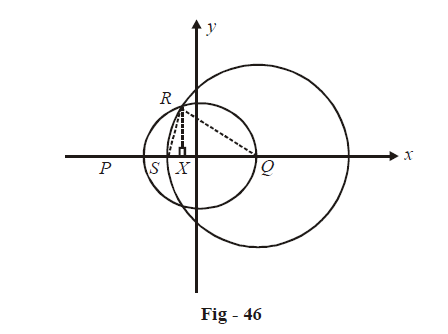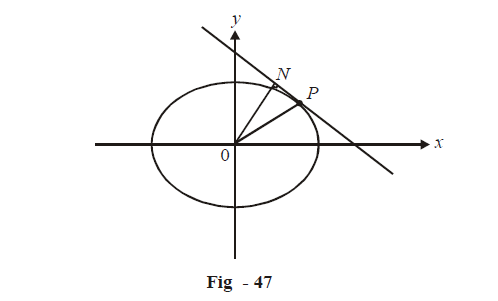# Applications of Derivatives Set 5

Go back to  'SOLVED EXAMPLES'

Learn from the best math teachers and top your exams

• Live one on one classroom and doubt clearing
• Practice worksheets in and after class for conceptual clarity
• Personalized curriculum to keep up with school

Example - 10

The circle  $${x^2} + {y^2} = 1$$  cuts the x-axis at P and Q. Another circle with center at Q and variable radius intersects the first circle at R above the x-axis and the line segment PQ at S. Find the maximum area of $$\Delta QSR.$$

Solution: The situation described in the question has been translated into the diagram below; observe it carefully:The variable here is the radius of the circle centered at Q; let it be r. We need to express the area A of $$\Delta \,QSR.$$ in terms of r. Observe $$\Delta \,QSR$$ carefully in the diagram. QR and QS are known (both are equal to r). We need to find RX (the height) in terms of R. We can do this by finding the co-ordinates of R:

\begin{align}&{x^2} + {y^2} = 1 \\{\left( {x - 1} \right)^2} & + {y^2} = {r^2}\,\,\,\,\,\,\,\,\,\,\,\qquad \left\{\text{circle centered at Q} \right\}\end{align}

Solving these two equations, the co-ordinates of R turn out to be:

\begin{align}\left( {1 - \frac{{{r^2}}}{2},\frac{{r\sqrt {4 - {r^2}} }}{2}} \right)\end{align}

Therefore,

\begin{align}&\qquad RX = \frac{{r\sqrt {4 - {r^2}} }}{2}\\\\ &\Rightarrow\quad A = \frac{1}{2} \times QS \times RX\\\\&\qquad\quad \;= \frac{1}{4}{r^2}\sqrt {4 - {r^2}} \end{align}

For maximum area

\begin{align}&\frac{{dA}}{{dr}} = 0\,\,{\rm{and}}\,\,\frac{{{d^2}A}}{{d{r^2}}} < 0\\\\&\frac{{dA}}{{dr}} = \frac{1}{4}\left\{ {2r\sqrt {4 - {r^2}} - \frac{{{r^3}}}{{\sqrt {4 - {r^2}} }}} \right\}\\\\&\,\,\,\,\,\,\; \;= \frac{{8r - 3{r^3}}}{{4\sqrt {4 - {r^2}} }}\end{align}

This is 0 when

\begin{align}&\,\,\,\,\,\,\,\,\,\,\,\,\,\,\ 8r - 3{r^3} = 0\\\\& \Rightarrow \quad r = \sqrt {\frac{8}{3}} \end{align}

Verify that

${\left. {\frac{{{d^2}A}}{{d{r^2}}}} \right|_{r = \sqrt {\frac{8}{3}} }} < 0$

Therefore,

\begin{align}{r_{\max }} = \sqrt {\frac{8}{3}} \end{align} is a local maximum for A.

\begin{align}&{\left. {{A_{\max }} = \frac{1}{4}{r^2}\sqrt {4 - {r^2}} } \right|_{r = {r_{\max }}}}\\\\&\qquad\; = \frac{{4\sqrt 3 }}{9}\end{align}

Example - 11

P is a point on the ellipse \begin{align}\frac{{{x^2}}}{{{a^2}}} + \frac{{{y^2}}}{{{b^2}}} = 1\end{align}whose center is O and N is the foot of the perpendicular from O upon the tangent at P. Find the maximum area of $$\Delta OPN.$$

Solution:  Let P have the co-ordinates $$\left( {a\cos \theta ,\,\,b\sin \theta } \right)$$

${\left. {\frac{{dy}}{{dx}}} \right|_P} = \frac{{ - b}}{a}\cot \theta \qquad \text{ (refer to Ex - 9)}$

Equation of tangent:     $$bx\cos \theta + ay\sin \theta = ab$$      (Ex – 9 again)The distance of this tangent from the origin 0 is:

$ON = \frac{{ab}}{{\sqrt {{a^2}{{\sin }^2}\theta + {b^2}{{\cos }^2}\theta } }}$

To evaluate the area A of $$\Delta OPN,$$we need PN apart from ON:

\begin{align}&\quad\quad P{N^2} = O{P^2} - O{N^2}\\\\&\qquad\qquad\;= \left( {{a^2}{{\cos }^2}\theta + {b^2}{{\sin }^2}\theta } \right) - \frac{{{a^2}{b^2}}}{{{a^2}{{\sin }^2}\theta + {b^2}{{\cos }^2}\theta }}\\\\&\qquad\qquad\; = \frac{{\left( {{a^4} + {b^4}} \right){{\sin }^2}\theta {{\cos }^2}\theta + {a^2}{b^2}\left( {{{\sin }^4}\theta + {{\cos }^4}\theta } \right) - {a^2}{b^2}}}{{{a^2}{{\sin }^2}\theta + {b^2}{{\cos }^2}\theta }}\\\\&\qquad\qquad\; = \frac{{\left( {{a^4} + {b^4} - 2{a^2}{b^2}} \right){{\sin }^2}\theta {{\cos }^2}\theta }}{{{a^2}{{\sin }^2}\theta + {b^2}{{\cos }^2}\theta }}\\\\ &\Rightarrow\quad \,PN = \frac{{\left( {{a^2} - {b^2}} \right)\sin \theta \cos \theta }}{{\sqrt {{a^2}{{\sin }^2}\theta + {b^2}{{\cos }^2}\theta } }}\\\\ &\Rightarrow \qquad A = \frac{1}{2} \times PN \times ON\\\\&\quad\qquad \quad= \frac{{ab\left( {{a^2} - {b^2}} \right)\sin \theta \cos \theta }}{{2\left( {{a^2}{{\sin }^2}\theta + {b^2}{{\cos }^2}\theta } \right)}}\\\\&\quad\qquad \quad= \frac{{ab\left( {{a^2} - {b^2}} \right)}}{{2\left( {{a^2}\tan \theta + {b^2}\cot \theta } \right)}}\,\,\,\,\,\,\,\,\,\,\,\,\,\,\,\,\,\,\,\,\,\,\,\,\,\,\,\,\,\,\,\,\,\,\,\,...{\rm{ }}\left( i \right)\end{align}

For A to be maximum, the denominator in (i) should be minimum i.e. we can minize:

$D\left( \theta \right) = {a^2}\tan \theta + {b^2}\cot \theta$

For $$D\left( \theta \right)$$ to be minimum:

\begin{align}&\qquad\;\;\frac{{d\left( {D\left( \theta \right)} \right)}}{{d\theta }} = 0\,\,\,and\,\,\frac{{{d^2}\left( {D\left( \theta \right)} \right)}}{{d{\theta ^2}}} > 0\\\\ &\Rightarrow\quad \frac{{d\left( {D\left( \theta \right)} \right)}}{{d\theta }} = {a^2}{\sec ^2}\theta - {b^2}\,\cos {\sec ^{2\,}}\theta = 0\\\\& \Rightarrow\quad \tan \theta = \pm \frac{b}{a}\\\end{align}

Verify that \begin{align}{\left. {\frac{{{d^2}\left( {D\left( \theta \right)} \right)}}{{d{\theta ^2}}}} \right|_{\tan \theta = \frac{b}{a}}} > 0\end{align}

\begin{align} &\Rightarrow \quad D\left( \theta \right)\text{has a local minimum at}\tan \theta = \frac{b}{a}\\\\&{\left. { \Rightarrow \quad{D_{\min }}\left( \theta \right) = {a^2}\tan \theta + {b^2}\cot \theta } \right|_{\tan \theta = \frac{b}{a}}} = 2ab\\\\ &\Rightarrow \quad {A_{\max }} = \frac{{ab\left( {{a^2} - {b^2}} \right)}}{{2{D_{\min }}}} = \frac{{{a^2} - {b^2}}}{4}\end{align}

Learn from the best math teachers and top your exams

• Live one on one classroom and doubt clearing
• Practice worksheets in and after class for conceptual clarity
• Personalized curriculum to keep up with school

0